# Gini Index For Decision Trees

Decision trees are often used while implementing machine learning algorithms. The hierarchical structure of a decision tree leads us to the final outcome by traversing through the nodes of the tree. Each node consists of an attribute or feature which is further split into more nodes as we move down the tree.

Learn Algorithmic Trading: A Step By Step Guide
Making A Career In Algorithmic Trading

But how do we decide which attribute/feature should be placed at the root node, which features will act as internal nodes or leaf nodes? To decide this, and how to split the tree, we use splitting measures like Gini Index, Information Gain, etc.

In this blog, we will learn how the Gini Index can be used to split a decision tree.

We will go through the following topics in this blog:

Before starting with the Gini Index, let us first understand what splitting is and what are the measures used to perform it.

## What are splitting measures?

With more than one attribute taking part in the decision-making process, it is necessary to decide the relevance and importance of each of the attributes, thus placing the most relevant at the root node and further traversing down by splitting the nodes. As we move further down the tree, the level of impurity or uncertainty decreases, thus leading to a better classification or best split at every node. To decide the same, splitting measures such as Information Gain, Gini Index, etc. are used.

## What is Information Gain?

Information Gain is used to determine which feature/attribute gives us the maximum information about a class. It is based on the concept of entropy, which is the degree of uncertainty, impurity or disorder. It aims to reduce the level of entropy starting from the root node to the leave nodes.

Formula for Entropy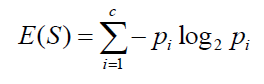p’, denotes the probability and E(S) denotes the entropy. Entropy is not preferred due to the ‘log’ function as it increases the computational complexity.

## What is Gini Index?

Gini index or Gini impurity measures the degree or probability of a particular variable being wrongly classified when it is randomly chosen. But what is actually meant by ‘impurity’? If all the elements belong to a single class, then it can be called pure. The degree of Gini index varies between 0 and 1, where 0 denotes that all elements belong to a certain class or if there exists only one class, and 1 denotes that the elements are randomly distributed across various classes. A Gini Index of 0.5 denotes equally distributed elements into some classes.

Formula for Gini Index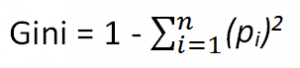where pi  is the probability of an object being classified to a particular class.

While building the decision tree, we would prefer choosing the attribute/feature with the least Gini index as the root node.

Let’s understand with a simple example of how the Gini Index works.

## Example of Gini Index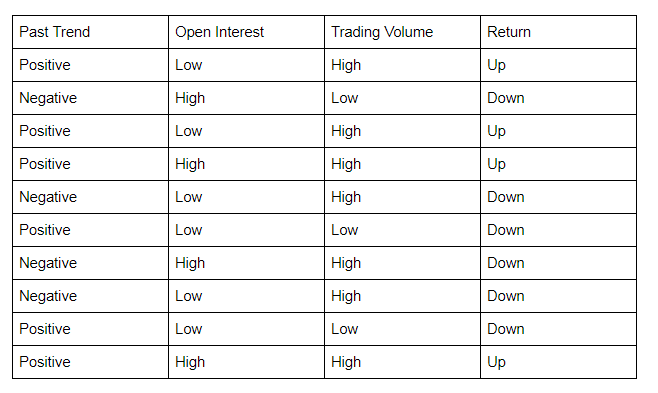### Let’s start by calculating the Gini Index for ‘Past Trend’.

P(Past Trend=Positive): 6/10

P(Past Trend=Negative): 4/10

If (Past Trend = Positive & Return = Up), probability = 4/6

If (Past Trend = Positive & Return = Down), probability = 2/6

Gini index = 1 - ((4/6)^2 + (2/6)^2) = 0.45

If (Past Trend = Negative & Return = Up), probability = 0

If (Past Trend = Negative & Return = Down), probability = 4/4

Gini index = 1 - ((0)^2 + (4/4)^2) = 0

Weighted sum of the Gini Indices can be calculated as follows:

Gini Index for Past Trend = (6/10)0.45 + (4/10)0 = 0.27

### Calculation of Gini Index for Open Interest

P(Open Interest=High): 4/10

P(Open Interest=Low): 6/10

If (Open Interest = High & Return = Up), probability = 2/4

If (Open Interest = High & Return = Down), probability = 2/4

Gini index = 1 - ((2/4)^2 + (2/4)^2) = 0.5

If (Open Interest = Low & Return = Up), probability = 2/6

If (Open Interest = Low & Return = Down), probability = 4/6

Gini index = 1 - ((2/6)^2 + (4/6)^2) = 0.45

Weighted sum of the Gini Indices can be calculated as follows:

Gini Index for Open Interest = (4/10)0.5 + (6/10)0.45 = 0.47

### Calculation of Gini Index for Trading Volume

If (Trading Volume = High & Return = Up), probability = 4/7

If (Trading Volume = High & Return = Down), probability = 3/7

Gini index = 1 - ((4/7)^2 + (3/7)^2) = 0.49

If (Trading Volume = Low & Return = Up), probability = 0

If (Trading Volume = Low & Return = Down), probability = 3/3

Gini index = 1 - ((0)^2 + (1)^2) = 0

Weighted sum of the Gini Indices can be calculated as follows:

Gini Index for Trading Volume = (7/10)0.49 + (3/10)0 = 0.34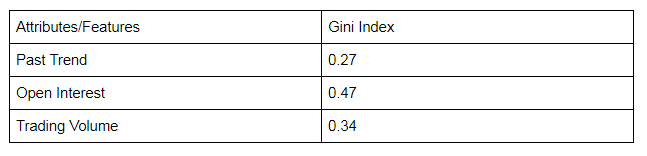From the above table, we observe that ‘Past Trend’ has the lowest Gini Index and hence it will be chosen as the root node for how decision tree works.

We will repeat the same procedure to determine the sub-nodes or branches of the decision tree.

We will calculate the Gini Index for the ‘Positive’ branch of Past Trend as follows: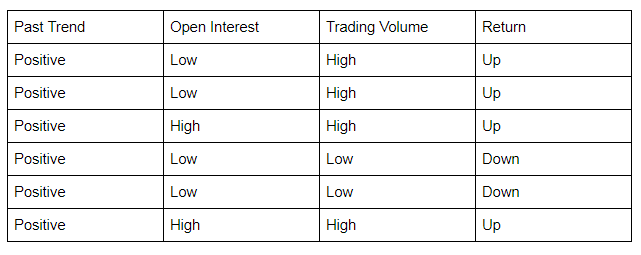### Calculation of Gini Index of Open Interest for Positive Past Trend

P(Open Interest=High): 2/6

P(Open Interest=Low): 4/6

If (Open Interest = High & Return = Up), probability = 2/2

If (Open Interest = High & Return = Down), probability = 0

Gini index = 1 - (sq(2/2) + sq(0)) = 0

If (Open Interest = Low & Return = Up), probability = 2/4

If (Open Interest = Low & Return = Down), probability = 2/4

Gini index = 1 - (sq(0) + sq(2/4)) = 0.50

Weighted sum of the Gini Indices can be calculated as follows:

Gini Index for Open Interest = (2/6)0 + (4/6)0.50 = 0.33

### Calculation of Gini Index for Trading Volume

If (Trading Volume = High & Return = Up), probability = 4/4

If (Trading Volume = High & Return = Down), probability = 0

Gini index = 1 - (sq(4/4) + sq(0)) = 0

If (Trading Volume = Low & Return = Up), probability = 0

If (Trading Volume = Low & Return = Down), probability = 2/2

Gini index = 1 - (sq(0) + sq(2/2)) = 0

Weighted sum of the Gini Indices can be calculated as follows:

Gini Index for Trading Volume = (4/6)0 + (2/6)0 = 0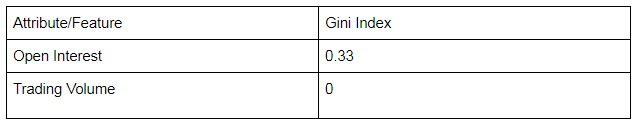We will split the node further using the ‘Trading Volume’ feature, as it has the minimum Gini index.

Learn how to make a decision tree to predict the markets and find trading opportunities using AI techniques with our Quantra course.

## Conclusion

Gini Index, unlike information gain, isn’t computationally intensive as it doesn’t involve the logarithm function used to calculate entropy in information gain, which is why Gini Index is preferred over Information gain.

You can learn more about different splitting measures including Gini Index, information gain, etc. in the course on Decision Trees.

Disclaimer: All data and information provided in this article are for informational purposes only. QuantInsti® makes no representations as to accuracy, completeness, currentness, suitability, or validity of any information in this article and will not be liable for any errors, omissions, or delays in this information or any losses, injuries, or damages arising from its display or use. All information is provided on an as-is basis.